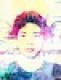VBA 的 `Function` 就像一般程式語言的函數，可傳入各種參數，進行自訂的運算，並將計算結果傳回。

VBA 的 `Sub``Function` 類似，可傳入各種參數並進行運算，但是沒有傳回值（沒有辦法傳回計算結果）。

## 子程序（`Sub`）

```Sub Hello()
MsgBox ("Hello, world!")
End Sub```

### 輸入參數

```' 自行定義的子程序
Sub mySub(x As Integer, y As Integer)
MsgBox ("x + y = " & x + y)
End Sub```

```Sub hello()
' 呼叫 mySub 子程序
mySub 1, 2
End Sub```

### 預設參數值

```' 自行定義的子程序
Sub mySub2(Optional x As Integer = 3, Optional y As Integer = 4)
MsgBox ("x + y = " & x + y)
End Sub```

```Sub hello()
' 計算 3 + 4
mySub2

' 計算 1 + 4
mySub2 1

' 計算 1 + 2
mySub2 1, 2
End Sub```

### 傳值與傳參考呼叫

```' 自行定義的子程序
Sub mySub3(x As Integer)
x = x + 1
End Sub```

```Sub Hello()
Dim val As Integer
val = 5
mySub3 val
MsgBox val
End Sub```

```' 傳值呼叫的子程序
Sub mySub3(ByVal x As Integer)
x = x + 1
End Sub```

```' 傳參考呼叫的子程序
Sub mySub3(ByRef x As Integer)
x = x + 1
End Sub```

### 8 留言

1.#### Caspar

感謝您的用心提供VBA教學，對我很有幫助!

2.#### Amity

感受到您的用心，在您的部落格裏學到不少東西。謝謝!

3.#### Sum

你好, 我想在做一個macro,
1)在Table-A, 選取C2的文字/數字,
2)然後在Table-B上搜尋和Table-A,C2相同的字,
3)然後在Table-B的行數(row)(和C2文字相同的行數)
,最後一個沒有資料的欄位(column)的儲存格,
4)將Table-A, A2的數字, copy寫在Table-B, 最後一個沒有資料的欄位(column)的儲存格.

煩請大大幫忙以下可以怎樣改, 謝謝.
以下”Cells.Find(What:=”BB”, _”,這個”BB”是每次都不同的,

Sub Macro1()
Application.ScreenUpdating = False

Range(“C2”).Select
Selection.Copy
Windows(“Table A.xlsx”).Activate
Cells.Find(What:=”BB”, _
After:=ActiveCell, _
LookIn:=xlFormulas, _
LookAt:=xlPart, _
SearchOrder:=xlByRows, _
SearchDirection:=xlNext, _
MatchCase:=False, _
MatchByte:=False, _
SearchFormat:=False).Activate

Application.CutCopyMode = False
Windows(“Table B.xlsm”).Activate
Range(“A2”).Select
Selection.Copy
Windows(“Table A.xlsx”).Activate
‘Range(“D19”).Select
‘ActiveSheet.Paste
‘Application.CutCopyMode = False
‘Windows(“Table B.xlsm”).Activate
Application.ScreenUpdating = True
End Sub

4.#### Stanley

5.#### CCLIU

非常實用得網站，謝謝您

6.#### Patrick

感受到您的用心，在您的部落格裏增長不少實力，很感謝您!

7.#### youshan

您好，朋友推薦我學 VBA，找到您的網站，覺得非常幸運，感謝您：）
想請問：
Sub hello()
‘ 計算 3 + 4
mySub2

‘ 計算 1 + 4
mySub2 1

‘ 計算 1 + 2
mySub2 1, 2
End Sub

請問如果要「計算 3+2」 呢？我的認知是「mySub2 1」的意思是把「3,4」中的第一個替換為「1」，但如果我只想替換掉「4」，可是想保留「2」呢？謝謝您！

8.#### youshan

您好，

朋友推薦我學 VBA，發現您的網站寫的非常簡明易懂，感謝您！

想請問：

Sub hello()
‘ 計算 3 + 4
mySub2

‘ 計算 1 + 4
mySub2 1

‘ 計算 1 + 2
mySub2 1, 2
End Sub

請問如果我是想要「3+7」呢？也就是，我想替換掉「3,4」中的第二個數字，但保留第一個數字 “3”。謝謝您！